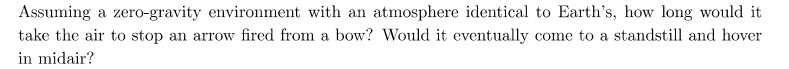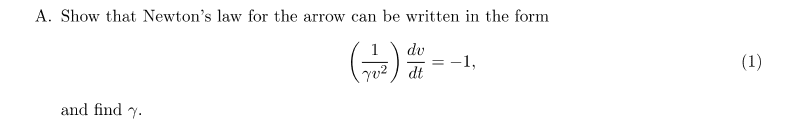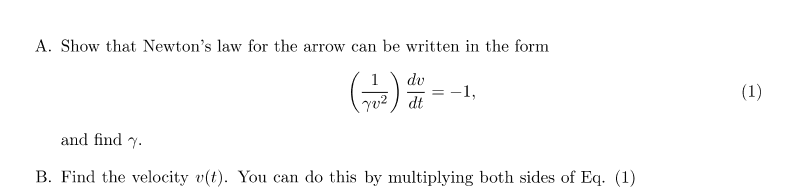# Need Help to Understand an Equation for Air Resistance

• Some1WhoNeedsHelp
The time integral is undefined because it is impossible to calculate the time integral for a function that is undefined at one point.

#### Some1WhoNeedsHelp

Homework Statement
Show That Newton's law for the arrow can be writteb in the form
Relevant Equations
F=maI don't understand what the gamma letter in the equation is supposed to represent.

What do you get when you multiply both sides of the equation with ##-\gamma v^2##? Can you interpret what it says?

kuruman said:
What do you get when you multiply both sides of the equation with ##-\gamma v^2##? Can you interpret what it says?
1=-gamma v^2?
I also need to find gamma, So I need to realize what the letter supposed to represent
I looked at the formula sheet and didn't find it there

Last edited:
Some1WhoNeedsHelp said:
1=-gamma v^2?
Nope. You get $$\frac{dv}{dt}=-\gamma~v^2.$$If I tell you that ##\gamma## is just a positive constant, can you interpret what this equation is saying to you regarding the motion of the arrow?

•Some1WhoNeedsHelp
kuruman said:
Nope. You get $$\frac{dv}{dt}=-\gamma~v^2.$$If I tell you that ##\gamma## is just a constant, can you interpret what this equation is saying to you regarding the motion of the arrow?
it's a function of speed relative to the time it represents
The change in speed is divided by the change in time.

So does it represent the location of the arrow?
Is that got something to do with the function:

X=X0+VT+(1/2)AT2

?

Some1WhoNeedsHelp said:
it's a function of speed relative to the time it represents
The change in speed divided by the change in time

Is that got something to do with the function:

X=X0+VT+(1/2)AT2

?
Nope. What you wrote down is the position of an object as a function of time under constant acceleration. Is the acceleration constant in this case? As a matter of fact look at the equation. Can you figure out an expression for the acceleration? Hint: How is the acceleration defined in terms of the velocity?

•Some1WhoNeedsHelp
kuruman said:
Nope. What you wrote down is the position of an object as a function of time under constant acceleration. Is the acceleration constant in this case? As a matter of fact look at the equation. Can you figure out an expression for the acceleration? Hint: How is the acceleration defined in terms of the velocity?
a = dv/dt

acceleration is meter/(seconds)2

and v2 is (meter)2/(seconds)2
So gamma must be meter

and using Newton's law

ma=(Cd*ρ*A*V2)/2

and since a = dv/dt

Gamma=-(Cd*ρ*A)/2m

Is that correct?

Last edited:
Gamma is a positive constant. The motion of the arrow is opposed by air resistance (it's like friction). So in the equation ##\dfrac{dv}{dt}=-\gamma~v^2##, the left-hand side is a negative number because the speed ##v## decreases with time. Can you answer the questions posed by the problem?

kuruman said:
Gamma is a positive constant. The motion of the arrow is opposed by air resistance (it's like friction). So in the equation ##\dfrac{dv}{dt}=-\gamma~v^2##, the left-hand side is a negative number because the speed ##v## decreases with time. Can you answer the questions posed by the problem?

So should I do Absolute value?

F=ma
F=(Cd*ρ*A*V2)/2
ma=(Cd*ρ*A*V2)/2
a=(Cd*ρ*A*V2)/2m
a = dv/dt
dv/dt = -γV2
-γV2=(Cd*ρ*A*V2)/2m
γ=|-(Cd*ρ*A)/2m|

Last edited:
Look
dv/dt = -γV2
a = dv/dt = -γV2
F = ma = -mγV2
The force is in the same direction as the acceleration and both of them are opposite to the direction of motion. The constant γ is positive. You don't need the absolute value if you handle the signs correctly.

kuruman said:
Look
dv/dt = -γV2
a = dv/dt = -γV2
F = ma = -mγV2
The force is in the same direction as the acceleration and both of them are opposite to the direction of motion. The constant γ is positive. You don't need the absolute value if you handle the signs correctly.
Because the force is opposite to direction of motion, the equation needs to be negative?

F=-(Cd*ρ*A*V2)/2=-mγV2?

so

γ=(Cd*ρ*A)/2m

Last edited:
Some1WhoNeedsHelp said:
F=(Cd*ρ*A*V2)/2=-mγV2?
Is that correct?
No, it is not correct because it is self contradictory.
The magnitude |F| of the drag force is positive and one can write
|F|=(Cd*ρ*A*V2)/2=mγV2.
If one wants to write Newton's second law, one must write
F= m dv/dt = -(Cd*ρ*A*V2)/2 = -mγV2.

•Some1WhoNeedsHelp
kuruman said:
No, it is not correct because it is self contradictory.
The magnitude |F| of the drag force is positive and one can write
|F|=(Cd*ρ*A*V2)/2=mγV2.
If one wants to write Newton's second law, one must write
F= m dv/dt = -(Cd*ρ*A*V2)/2 = -mγV2.
so
γ=(Cd*ρ*A)/2m
?

Yes.

•Some1WhoNeedsHelp
kuruman said:
Yes.
yes!

But since γ is positive I have a new problem

I am trying to calculate the V

so I multiply by "dt" and do integral

∫(1/γV2) dv=∫dt

(v/γ)*(-1/v)=t

(-1/γ)=t

So time is negative

Time is not negative. You forgot the limits of integration. Assume that at ##t=0## the arrow has velocity ##v_0## and some later time ##t## it has some other velocity ##v##. Set the limits of integration, then integrate.

•Some1WhoNeedsHelp
kuruman said:
Time is not negative. You forgot the limits of integration. Assume that at ##t=0## the arrow has velocity ##v_0## and some later time ##t## it has some other velocity ##v##. Set the limits of integration, then integrate.
v0v(1/γV2) dv=∫0tdt

(v0/γ)*(-1/v0)-(v/γ)*(-1/v)=-t

(-1/γ)-(-1/γ)=t

0=t

correct?

Some1WhoNeedsHelp said:
v0v(1/γV2) dv=∫0tdt

(v0/γ)*(-1/v0)-(v/γ)*(-1/v)=-t

(-1/γ)-(-1/γ)=t

0=t

correct?
I have no idea how you get that result from the integration. What is the integral (1/x2).dx?
Try again, taking one small step at a time so that we can see where your error is.

haruspex said:
I have no idea how you get that result from the integration. What is the integral (1/x2).dx?
Try again, taking one small step at a time so that we can see where your error is.
The integral of (1/x2).dx is (-1/x)

so
(-1/γv0)-(-1/γv)=-t

Some1WhoNeedsHelp said:
The integral of (1/x2).dx is (-1/x)

so
(-1/γv0)-(-1/γv)=-t
That looks better.
But please use parentheses appropriately. What you have written means (1/γ)v, where you mean 1/(γv). And it would have been more natural to have set the bounds the other way up, ##\int_{v_0}^v, \int_0^t##.

•Some1WhoNeedsHelp and berkeman
haruspex said:
That looks better.
But please use parentheses appropriately. What you have written means (1/γ)v, where you mean 1/(γv). And it would have been more natural to have set the bounds the other way up, ##\int_{v_0}^v, \int_0^t##.
-1/(γvt)+1/(γv0)=t

I know

γ,V0

so I get - 1 equation with 2 unknown things (Vt,t)

I would assume Vt should be equal to 0 since the drag force will stop the arrow eventually but the question asks me to calculate the value of Vt using the equation.

I also know η, Cd , A, ρ and the length of the arrow, if that helps.

Some1WhoNeedsHelp said:
but the question asks me to calculate the value of Vt
No, it asks you to find the value of t.

haruspex said:
No, it asks you to find the value of t.Oh wait, Is that mean I need to find an expression of V depending on t?

So am I right about the drag force will stop the arrow eventually despite being the only force?

Last edited:
Some1WhoNeedsHelp said:
Is that mean I need to find an expression of V depending on t?
You did that in post #21
Some1WhoNeedsHelp said:
So am I right about the drag force will stop the arrow eventually despite being the only force?
That's for you to figure out. In fact it asks you to find how long it will take:
https://www.physicsforums.com/attachments/help2-png.318235/

•Some1WhoNeedsHelp
haruspex said:
You did that in post #21

That's for you to figure out. In fact it asks you to find how long it will take:
https://www.physicsforums.com/attachments/help2-png.318235/
Alright so according to the equation I created from post #21

-1/(γvt)+1/(γv0)=t

-v0+vt=-γvtv0t

vt+γvtv0t=v0

vt(1+γv0t)=v0

vt=v0/(1+γv0t)

So as time keeps increasing the vt will decrease to the point the speed of the arrow will become neglectable, so the arrow will stop

Some1WhoNeedsHelp said:
vt will decrease to the point the speed of the arrow will become neglectable, so the arrow will stop
How long will it take to stop, according to the formula?

I found someone in real life who help me to better understand the subject.
I realize now that the arrow won't stop as long as only drag force is active and gamma is 1/meter

Still thanks to both of you.

Last edited:
Some1WhoNeedsHelp said:
I found someone in real life who help me to better understand the subject.
I realize now that the arrow won't stop as long as only drag force is active and gamma is 1/meter

Still thanks to both of you.
We too exist in the "real life" plane. Have we not been helping you understand?

You can find an answer quite easily using power (energy loss per unit time) considerations. The integral of power over time is energy loss in this case. If you can find an expression for energy loss as a function of time, you can find how long it takes to lose ##\frac{1}{2}mv_0^2.##

Last edited:
•Some1WhoNeedsHelp
kuruman said:
We too exist in the "real life" plane. Have we not been helping you understand?

You can find an answer quite easily using power (energy loss per unit time) considerations. The integral of power over time is energy loss in this case. If you can find an expression for energy loss as a function of time, you can find how long it takes to lose ##\frac{1}{2}mv_0^2.##
you help me a lot to understand too, even with this guy help I wouldn't be able to understand what he tries to explain to me if not for you two

That is why I am thanking you
Let me just upload my homework and I will solve this

Some1WhoNeedsHelp said:
you help me a lot to understand too, even with this guy help I wouldn't be able to understand what he tries to explain to me if not for you two

That is why I am thanking you
Let me just upload my homework and I will solve this
•